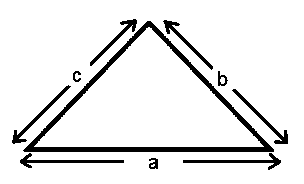# Perimeter of a Triangle Formula

• Perimeter of a triangle is the total length of the sides of a triangle. The sides of the triangle may be equal or unique.
• If all the three sides are same, then it is known as an equilateral triangle.
• It is an isosceles triangle if two of the sides are equal.• For a triangle to exist certain conditions need to be met. For any triangle, one of the following three conditions must be true.
• a + b > c
• b + c > a
• c + a > b

Now, the formula for the Perimeter of a Triangle is given as,

$\large Perimeter\;of\;a\;Triangle=a+b+c$

Where,
a, b, c are the sides of the triangle.

### Solved Examples

Question 1: Find the perimeter of a triangle whose sides are of  6 cm, 8 cm and 12 cm.

Solution:

Given,
a = 6 cm
b = 8 cm
c =  12 cm

Perimeter of a Triangle = a + b + c
= 6 + 8 + 12
= 26 cm

Question 2: Find the perimeter of an equilateral triangle whose side is  6 cm.

Solution:

Being an equilateral triangle, all the sides are equal.

Thus, a = 6

Perimeter of an equilateral triangle= a + b + c = a + a + a = 3a
= 3 x a

= 3 x 6
= 18 cm

Question 3: Find the perimeter of an isosceles triangle whose equal sides measure 6 cm and the third side is 8 cm.

Solution:

Being an isosceles triangle, two sides are equal.

Thus, a = 6, b = 6 and c = 8

Perimeter of an isosceles triangle= a +b + c = a + a + c = 2a + c
= 2 x 6 + 8

= 12 + 8
= 20 cm

For more: Math Formulas.